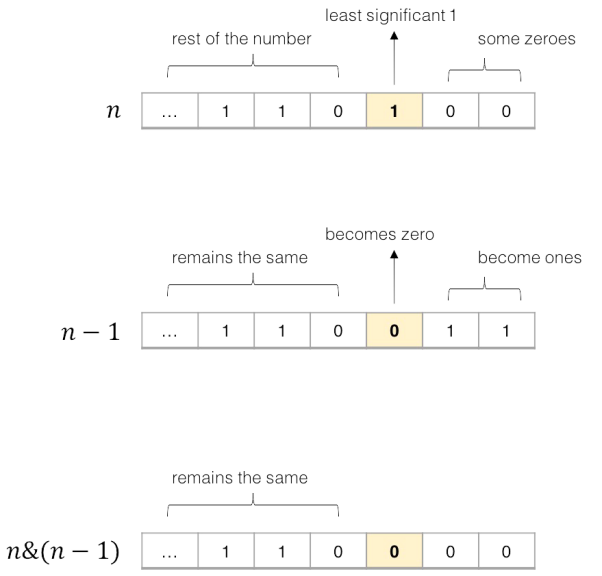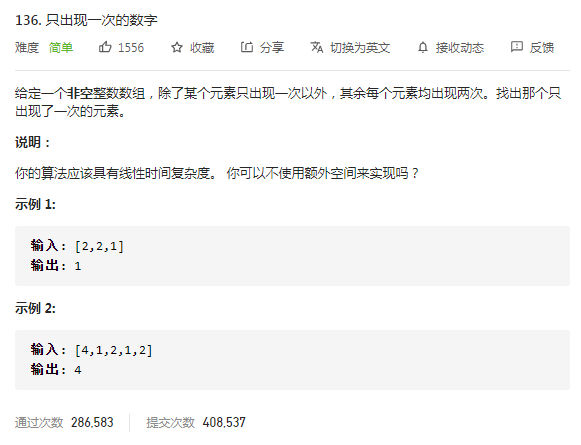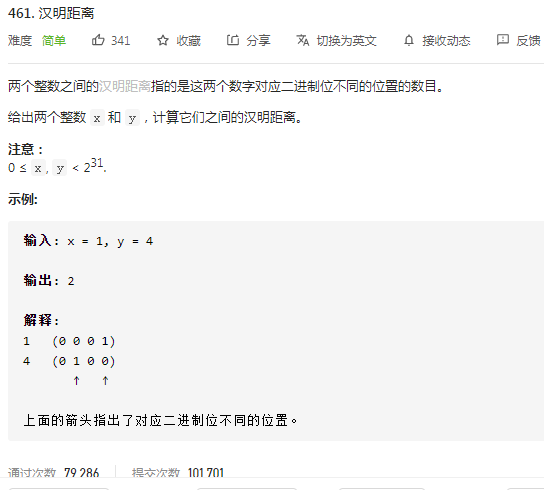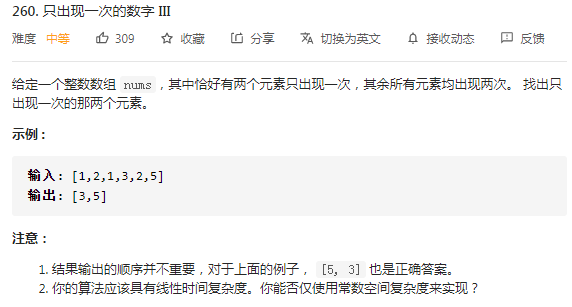# vcjmhg 的个人博客

## 常见的位操作及其应用# 有趣的操作

## 1. 大小写字母转换

1. 利用 `或操作` 和空格将英文字母转成小写
``````1('a' | ' ') = 'a';
2('A' | ' ') = 'a';
``````
1. 利用与运算 `&` 和下划线将英文字符转换成大写
``````1('b' & '_') = 'B';
2('B' & '_') = 'B';
``````
1. 利用异或运算 `^` 和空格进行英文字符大小写互换
``````1('d' ^ ' ') = 'D';
2('D' ^ ' ') = 'd'
``````

PS:上述技巧能够产生奇特效果的原因在于字符类型的数据都是通过 ASCII 进行编码的，字符本身其实就是数字，而刚好这些字符对应的数字通过位运算符就可以得到正确结果，此处就不展开来说了。

## 2. 判断两个数是否异号

``````1int x = -1, y = 2;
2boolean f = ((x ^ y) < 0); // true 两个int类型数据进行异或运算小于零证明异号
3
4int x = 1, y = 2;
5boolean f = ((x ^ y) < 0); // false 两个int类型数据进行疑惑运算大于零证明同好
6
``````

PS： 这个操作在我们判断两个数异号的时候非常有用，一方面运算效率较高，另一方面可以减少 if else 分支的使用。其背后的原理主要是一个正数补码的符号位和一个负数补码的符号位肯定想法，经过疑惑运算后，最后符号位结果肯定是 `1`(代表负数)。

## 3. 移除最后一位"1"

``````1byte n = 10;
2// n的二进制表示为: 0 0 0 0 1 0 1 0
3//       异或运算  ^
4//n-1的二进制表示为:0 0 0 0 1 0 0 1
5n & (n-1); //结果为:0 0 0 0 1 0 0 0
``````PS： 这个操作特别常用，在好多 leetcode 题目中都有涉及，比如用来判断一个数的二进制数中 1 的个数。

## 4. 获取最后一个 1

``````1byte n = 10;
2//结果为:0 0 0 0 0 0 1 0
3(n & (n-1)) ^ n;
``````

PS: 该操作刚好和[操作 3](# 3. 移除最后一位"1")相反，主要是为了获取数字 n 的二进制表示中的最后一个 1.该操作通常会用在位标记的时候使用（可参考[汉明距离](#2. 汉明距离)）。

## 5. 异或运算的简单性质

``````1a=0^a=a^0
2
30=a^a
``````

PS:这个性质在我们判断两个数是否相同的时候非常常用。

# 应用

## 1. 只出现一次的数字``````1public int singleNumber(int[] nums) {
2        int result = 0;
3        for (int i =0;i<nums.length;i++){
4            result ^=nums[i];
5        }
6        return result;
7    }
``````

## 2. 汉明距离``````1 public int hammingDistance(int x, int y) {
2    x = x ^ y;
3    int count = 0;
4    while (x != 0) {
5      x = x & (x - 1);
6      count++;
7    }
8    return count;
9  }
``````

## 1. 只出现一次的数字 III``````1int a[] = {1, 1, 3, 5, 2, 2}
``````

`0x0110`，第 1 位（由低向高，从 0 开始）就是 1。因此整个数组根据第 1 位是 0 还是 1 分成两组。

`````` 1a =1  0x0001  第一组
2
3a =1  0x0001  第一组
4
5a =3  0x0011  第二组
6
7a =5  0x0101  第一组
8
9a =2  0x0010  第二组
10
11a =2  0x0010  第二组
``````

`````` 1public int[] singleNumber(int[] nums) {
2    int xorVal = 0;
3    for (int num : nums) {
4        xorVal ^= num;
5    }
6    // 获取最后一个 1
7    xorVal = (xorVal & (xorVal - 1)) ^ xorVal;
8
9    int res[] = new int;
10    //根据和xorVal的与运算结果不同进行分类
11    for (int num : nums) {
12        if ((num & xorVal) == 0) {
13            res ^= num;
14        } else {
15            res ^= num;
16        }
17    }
18    return res;
19}
``````